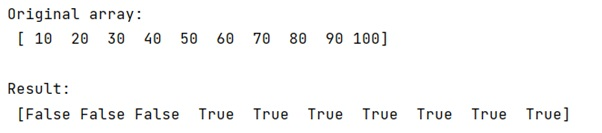# How to perform element-wise Boolean operations on NumPy arrays?

Learn, how to perform element-wise Boolean operations on NumPy arrays?
Submitted by Pranit Sharma, on January 15, 2023

NumPy is an abbreviated form of Numerical Python. It is used for different types of scientific operations in python. Numpy is a vast library in python which is used for almost every kind of scientific or mathematical operation. It is itself an array which is a collection of various methods and functions for processing the arrays.

## Performing element-wise Boolean operations on NumPy arrays

In programming, we sometimes use some specific values that only have two values, either True or False. These values are known as Boolean values.

Suppose that we are given a NumPy array that contains some integer values and we need to create a mask that masks elements with values that lie between a certain range.

For this purpose, we will use the simple OR operation which will help us to create a mask and filter out those values that lie in a specific range.

Let us understand with the help of an example,

## Python code to perform element-wise Boolean operations on NumPy arrays

```# Import numpy
import numpy as np

# Creating a numpy array
arr = np.array([10,20,30,40,50,60,70,80,90,100])

# Display original array
print("Original array:\n",arr,"\n")

# performing boolean operation on each element
res = (arr>30)

print("Result:\n",res)
```

Output:What's New (MCQs)

Top Interview Coding Problems/Challenges!

IncludeHelp's Blogs

Languages: » C » C++ » C++ STL » Java » Data Structure » C#.Net » Android » Kotlin » SQL
Web Technologies: » PHP » Python » JavaScript » CSS » Ajax » Node.js » Web programming/HTML
Solved programs: » C » C++ » DS » Java » C#
Aptitude que. & ans.: » C » C++ » Java » DBMS
Interview que. & ans.: » C » Embedded C » Java » SEO » HR
CS Subjects: » CS Basics » O.S. » Networks » DBMS » Embedded Systems » Cloud Computing
» Machine learning » CS Organizations » Linux » DOS
More: » Articles » Puzzles » News/Updates